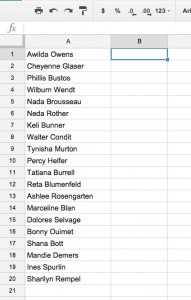There are times when you need to randomize students in a list, such as to call on students one at a time or if you want to randomly group students. A quick way to do that is to use Google Sheets. This isn’t limited to Google Sheets, it will also work in other spreadsheet programs.Put your list of names in the first column. In the second column, enter the following function:

`=rand()`

Then fill down the second column with that function. In a spreadsheet, formulas start with the equals sign (=). Formulas are used to calculate or assign a different value and store that value in the cell which contains the formula. For this example, we are going to use the rand() function.

A function is a command that will return a value based on its purpose. The rand() function returns a fractional random number between 0 and 1. So our formula in each cell beside the name will be set to a random number between 0 and 1 and will be used to randomize students. There is also the randbetween() function that will return numbers between any two sets of integers, but for our purpose rand() is all we need.

Once that is done, you’re ready to randomize. In the header for the second column, press the triangle menu and select Sort A-Z.Your names are now in random order. Every time you re-sort, your names will be in a different order because the rand() function will return a different random number. Why this works is that Google Sheets will keep the content on each row together.

You can use the third column to set them in groups (such as putting the students in groups of 4, in the 3 column, put a 1 in the first four spots, a 2 in the next four, and so on). I’m sure there are other ways of doing this, let me know in the comments!